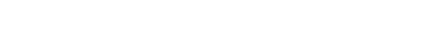# The VALUE Function Explained: Convert Text to Numbers

Written by co-founder Kasper Langmann, Microsoft Office Specialist.

In this tutorial, you learn how to use the ‘VALUE’ function in Excel.

The VALUE function convert numbers stored as text into number format.

Don’t worry if it sounds a bit complicated, I show you everything step-by-step.

Later, you’ll also see how this conversion is can be necessary for calculations and formulas to work properly.

As always, the best way to learn how these functions work is by looking at some examples. Let’s get right into it!

## Get your FREE exercise file

Before you start:

Throughout this guide, you need a data set to practice.

## How to use the ‘VALUE’ function

There are cases where numerical values are stored in cells as text – and we need to convert them to a number.

As you’re about to see, the ‘VALUE’ function brings much-needed relief to some real data quality headaches.When we type in ‘=VALUE’ the tooltip clearly tells us what the function does:

“Converts a text string that represents a number to a number”

The syntax is as simple as it gets. The only argument necessary is the text that needs to be converted.It’s not super common to have the need for the ‘VALUE’ function. But as with anything, there are exceptions to this!

Sometimes you need ‘VALUE’ when calculations – or various other formulas that rely on some value stored as text – do not work as intended. This can be a real headache.

For instance, when a part number – or product code – that a lookup formula relies on is not only stored as text but may even have trailing spaces.

“Trailing spaces?! But we can use the TRIM function to remove that!”. That’s true and great suggestion by the way! But, it won’t solve our problem as it won’t convert text to numbers.

And if our lookup table contains number value data, we still haven’t solved all our issues.

The ‘VALUE’ function fixes both issues in one step.

Let’s take a closer look!Our lookup table is based on product codes that are number format.

Thus, it is necessary that the data we will be indexing to this table will also be in number format.

However, it is not uncommon that raw data can come in many formats other than what we expect.

To learn how to solve this, I’ve setup an example for you. Here our raw data product codes are in text format with trailing spaces.

For further illustration, the following figure shows that we have cleansed our data using the ‘TRIM’ function first.

Then we’ve used the ‘VALUE’ function. Notice that at a glance, the data in all three columns appears the same.But the real issue becomes evident once we attempt to build our formula to index our data to the lookup table.

The only formula that works is the one in the ‘Lookup 3’ column.

This references the values in column C where we have used the ‘VALUE’ function.

The ‘VALUE’ function not only converted the text version of the product code data in the ‘Original Data’ column… It also removed the trailing spaces in the process!

The ‘TRIM’ function removed trailing spaces for us… but it didn’t address the issue of the original data being a text representation of our product codes. Because of this our formulas in the ‘Lookup 2’ column return errors.

Our ‘Lookup 1’ column formulas reference the original data.

This illustrates the basic point that numbers stored as text will not match actual number values in our lookup table.

## Conclusion: Wrapping up…

Now you know exactly why VALUE is so powerful 🙂

VALUE’s use is relatively specialized compared to many other functions in Excel (like VLOOKUP). But when you need it, it sure is one function worth keeping in your bag of tricks!